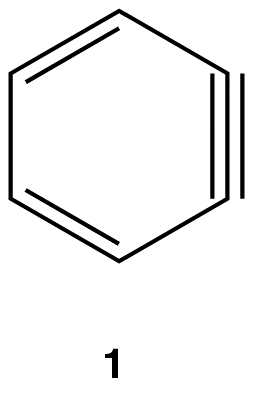# Benzyne

$$\newcommand{\vecs}{\overset { \rightharpoonup} {\mathbf{#1}} }$$ $$\newcommand{\vecd}{\overset{-\!-\!\rightharpoonup}{\vphantom{a}\smash {#1}}}$$$$\newcommand{\id}{\mathrm{id}}$$ $$\newcommand{\Span}{\mathrm{span}}$$ $$\newcommand{\kernel}{\mathrm{null}\,}$$ $$\newcommand{\range}{\mathrm{range}\,}$$ $$\newcommand{\RealPart}{\mathrm{Re}}$$ $$\newcommand{\ImaginaryPart}{\mathrm{Im}}$$ $$\newcommand{\Argument}{\mathrm{Arg}}$$ $$\newcommand{\norm}{\| #1 \|}$$ $$\newcommand{\inner}{\langle #1, #2 \rangle}$$ $$\newcommand{\Span}{\mathrm{span}}$$ $$\newcommand{\id}{\mathrm{id}}$$ $$\newcommand{\Span}{\mathrm{span}}$$ $$\newcommand{\kernel}{\mathrm{null}\,}$$ $$\newcommand{\range}{\mathrm{range}\,}$$ $$\newcommand{\RealPart}{\mathrm{Re}}$$ $$\newcommand{\ImaginaryPart}{\mathrm{Im}}$$ $$\newcommand{\Argument}{\mathrm{Arg}}$$ $$\newcommand{\norm}{\| #1 \|}$$ $$\newcommand{\inner}{\langle #1, #2 \rangle}$$ $$\newcommand{\Span}{\mathrm{span}}$$

Hydrocarbon 1 is called benzyne.Derivatives of 1 are called benzynes or arynes.

eg:If each triply bonded carbon atom in a benzyne molecule is sp-hybridized, as is typical of triply bonded carbon atoms, there would be severe angle strain in the molecule. It is more likely that each triply bonded carbon atom in a benzyne molecule is sp2-hybridized, in which case two sp2-hybridized orbitals that are not parallel to each other overlap laterally to form the pi bond that is not part of the loop of pi electrons (2).In either case, the pi bond in a benzyne molecule that is not part of the loop of pi electrons would be very weak, which is consistent with the observation that, despite being aromatic, benzynes are extremely unstable and reactive. If generated in isolation, benzynes undergo rapid dimerization.

eg:## Mechanism

Step 1:Step 2: